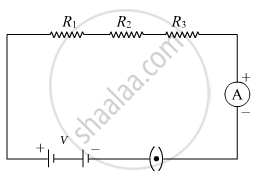# How will you infer with the help of an experiment that the same current flows through every part of the circuit containing three resistors R1, R2 and R3 in series connected to a battery of V volts? - Science

How will you infer with the help of an experiment that the same current flows through every part of the circuit containing three resistors R1, R2 and R3 in series connected to a battery of V volts?

#### Solution

Consider the given circuit in which a series combination of three resistors of resistances  R1, R2 and R3 are shown to be connected to a battery of V volts. An ammeter is also shown to be connected in the circuit. It can be inferred that the current remains the same in the circuit by placing the ammeter at different points in the same circuit i.e. At any point just after R1, R2, and R3. It is observed that the value of the current observed in the ammeter comes to be the same.Is there an error in this question or solution?